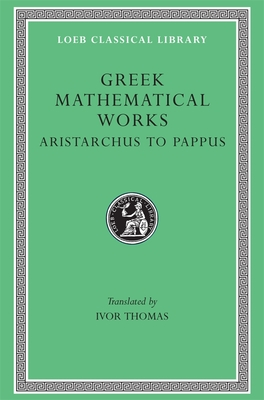### Greek Mathematical Works, Volume II (Hardcover)

Aristarchus to Pappus (Loeb Classical Library #362)

By Ivor Thomas (Translator)

Harvard University Press, 9780674993990, 704pp.

Publication Date: January 1, 1941

Other Editions of This Title:
Hardcover, Greek, Ancient (to 1453) (1/1/1939)

List Price: 28.00*
* Individual store prices may vary.

#### Description

The wonderful achievement of Greek mathematics is here illustrated in two volumes of selected mathematical works. Volume I (Loeb Classical Library no. 335) contains: The divisions of mathematics; mathematics in Greek education; calculation; arithmetical notation and operations, including square root and cube root; Pythagorean arithmetic, including properties of numbers; square root of 2; proportion and means; algebraic equations; Proclus; Thales; Pythagorean geometry; Democritus; Hippocrates of Chios; duplicating the cube and squaring the circle; trisecting angles; Theaetetus; Plato; Eudoxus of Cnidus (pyramid, cone); Aristotle (the infinite, the lever); Euclid.

Volume II contains: Aristarchus (distances of sun and moon); Archimedes (cylinder, sphere, cubic equations; conoids; spheroids; spiral; expression of large numbers; mechanics; hydrostatics); Eratosthenes (measurement of the earth); Apollonius (conic sections and other works); later development of geometry; trigonometry (including Ptolemy's table of sines); mensuration: Heron of Alexandria; algebra: Diophantus (determinate and indeterminate equations); the revival of geometry: Pappus.